Courses

# A/D and D/A Converters - Digital Electronics, Electronics and Experimental Methods, CSIR-NET Physic Physics Notes | EduRev

## Physics for IIT JAM, UGC - NET, CSIR NET

Created by: Akhilesh Thakur

## Physics : A/D and D/A Converters - Digital Electronics, Electronics and Experimental Methods, CSIR-NET Physic Physics Notes | EduRev

The document A/D and D/A Converters - Digital Electronics, Electronics and Experimental Methods, CSIR-NET Physic Physics Notes | EduRev is a part of the Physics Course Physics for IIT JAM, UGC - NET, CSIR NET.
All you need of Physics at this link: Physics

Digital­to­Analog Conversion

A digital­ to ­analog (D/A) converter (DAC) is a chip or circuit that converts a digital number into an analog voltage or current. D/As are used to control devices that require a range of control voltages or currents such as electro­acoustic transducers (speakers), some types of variable­speed motors, and many other applications where an analog signal output is required. The most common application is to [re]create waveforms from digital signals – for example in CD players.

D/A Converters

A D/A converter can be visualized as a circuit that adds up a number of voltages under the control of a digital signal. Each voltage can be turned on and off by an electronic switch which is controller by the digital input. The circuit below shows a 3-­bit D/A consisting of a summing ampliﬁer fed by three different voltages. Depending on which switches are closed, the output can range from 0 to 7 volts. By using digital signals to control the switches we can build a circuit whose output voltage is proportional to the digital value.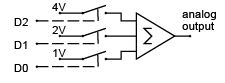D/A Converter Speciﬁcations

Many different Digital­to­Analog converters are commercially available, both as chips and as subsystems (modules, boards, etc). To select the right D/A converter it is necessary to understand D/A speciﬁcations. The most important specs are resolution and settling time. In some applications other speciﬁcations such as slew rate, linearity, monotonicity may also be important. There are also various types of digital and analog interfaces. Since different manufacturers use different deﬁnitions for some of these speciﬁcations it is important to check each manufacturer’s deﬁnitions when comparing devices.
The resolution (output step size) is given by the output voltage range divided by the number of possible output levels. An N ­bit DAC can output 2N different levels in 2N -1 steps. N ranges from 6 (for simple high­speed converters) up to 20 or more (for precision instrumentation).

The accuracy is the maximum difference between the output and the theoretical output. This is typically (but not always) this is approximately the same as the resolution.
The settling time is the time it takes the output to reach a percentage (e.g. 99%) of the ﬁnal value.
Values can range from hundreds of milliseconds for high­precision units to nanoseconds for devices used in high­frequenc y waveform generators.
The linearity is the maximum difference between the output and a linear interpolation between the minimum and maximum values. This is usually on the order of 1 / 2N .
The output is monotonic if an increase in the digital input always causes an increase in the analog out­ put. This is usually guaranteed.
There are various types of digital DAC interfaces.
Some devices have chip­select and address pins and can be easily interfaced to microcomputers as if they were peripheral chips. Other devices omit the registers and thus require additional logic to interface to a microprocessor.
There are various types of DAC output circuits.
Some are designed to generate a current (rather than a voltage) that is proportional to the digital input. Often the DAC is simply is a set of N switches and an accurate voltage divider network and an external voltage reference must be supplied.
Finally, there are different ways of manufacturing DAC devices. In addition to the standard monolithic ICs, there are also hybrid devices that mount IC chips together with a small number of discrete devices into an IC­like package. This allows the manufacturer to adjust (“trim”) each device during manufacture to obtain higher precision. There are also DAC modules in which a D/A converter built from discrete components is packaged in a sealed module. Hybrids and modules tend to be used for higher­performance devices.

Analog to Digital Converters

A controller often has to measure a physical quantity, for example temperature, pressure, force, etc.
A sensor, often called a transducer, is used to convert this physical quantity into an electrical signal (current or voltage). This electrical signal must then be converted into a binary number so that the digital controller can use it. An analog to digital (A/D) converter (ADC) performs this function.

Comparators

The simplest type of ADC is a comparator. As its name indicates, a comparator compares two analog inputs (say vin and vref ) and outputs a logic signal which is high if vin is greater than vref or low otherwise. Comparators are available as ICs, often with two or four units in one IC.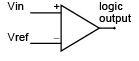The comparator can be considered to be a one­bit ADC since it’s output tells us whether the input voltage is above or below the reference voltage.
Although a comparator does not offer much precision, it is often sufﬁcient since for many applications it’s only necessary to determine whether some quantity is above or below some threshold. Comparators also form the basis of other types of A/D converters as will be described below

Types of A/D Converters

Flash Converters

A simple way to get better (more bits of) resolution is to use more comparators. As shown below for a 2­bit ﬂash converter we can use 2- 1 comparators, supplying them with reference voltages that are equally spaced over the desired conversion range. The other comparator inputs are connected to the input signal.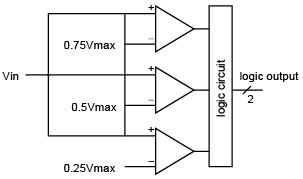All of the digital outputs connected to reference voltages below the input signal will be true and all of the outputs with reference signals above the input signal level will be false. The output logic circuit converts these 2- 1 binary values into an N ­bit number.

Successive Approximation Converters

Another approach is to use a D/A converter to generate the reference voltage and a single comparator to compare this voltage and the input. A digital circuit can step the D/A output up through the possible values until the comparator indicates that the reference signal is greater than the input signal. The digital input to the D/A would correspond to the voltage step that is the next­highest to the input signal. This approach would take up to 2N comparisons.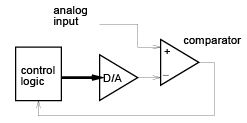A faster method is to use a binary search. The control circuit ﬁrst tests whether the analog input is greater than or less than half of the D/A output range. This ﬁxes the value of the most signiﬁcant bit.
Then the controller tests whether the value is greater than or less than the half­way point of the remaining range. This sets the next most­signiﬁcant bit. The process is repeated until the values of all of the bits are determined. The time required for this method is about N times the D/A settling time.
This method is slower than a ﬂash converter but reduces the number of comparators required. Successive approximation converters are probably the most common type of general­purpose ADCs.

Single­ and Dual­Slope Converters

A different approach, commonly used in highprecision low­speed ADCs, is to use the input signal to charge up a capacitor until the capacitor voltage reaches a ﬁxed threshold. A digital circuit measures the time required to reach this threshold by counting the number of pulses from a ﬁxed­frequency clock.
This approach is often used in slow, low­precision converters because very little hardware (one comparator and one digital output to reset the capacitor) is required.

One problem with this approach is that very accurate components are required to obtain precise results (e.g. the capacitors and resistors used in the integration). A slightly different approach is to use the input voltage to charge up the capacitor as before, but to do this for a ﬁxed time. Then the capacitor is discharged by a reference voltage and the digital control circuit measures the time required to completely discharge the capacitor. The ratio of the discharge time to the charging time is the ratio of the unknown to the known voltage. This dual­slope method is independent of the values of the integrator components because the same circuit is used for charging and discharging.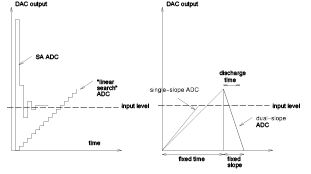This method can be quite slow since sufﬁcient conversion time must be allowed the counter must count up to 2N . However since this method is quite accurate it is often used in high precision (e.g. 16 ­bit) converters.

Voltage - ­to -­ Frequency Conversion

Yet another method of converting from a voltage to a number is to use the analog voltage to control the frequency of an oscillator. Various circuits and IC’s are available that oscillate at a frequency proportional to an input voltage. A counter can be used to count the number of pulses produced in a ﬁxed time and this becomes the digital output.
This approach is also relatively slow but also requires little hardware.

A/D Speciﬁcations

The most important speciﬁcations for an A/D converter, as for a D/A converter, are the resolution and accuracy (number of bits) and the conversion speed (in microseconds or milliseconds). Converters are available with resolutions from 6 (for some very fast ﬂash converters) to 18 or more (slow dual­slope models for high­precision applications).

Other speciﬁcations, such as linearity and monotonicity are similar to the speciﬁcations for D/A converters.

A/D converters also differ in the input voltage ranges that they can convert and in their digital output interfaces.

A/D Digital Interfaces

As with D/A converters, A/D converters are available as chips (monolithic or hybrids) that can be interface easily to a microcomputer as if they were standard peripheral chips. Most types of D/A converters need to be supplied with a periodic clock signal, a “start conversion” signal and they generate a “conversion complete” status signal when the conversion is done. The software that handles the A/D must give the command to start the conversion (e.g. by setting a control bit), and then wait until the status bit indicates that the conversion is complete before reading the result.

Successive­approximation ADCs often provide their outputs in a serial format, one bit at a time, as the conversion takes place.

Sample and Hold

If the analog signal changes while a conversion is taking place, the ADC could produce an incorrect result. A device called a sample­and­hold can be used before the ADC to hold the signal at the input to the ADC constant. This device samples the analog signal at its input for a short time and then holds that value ﬁxed at its output until the next sample is required.

The diagram below shows how the device works. An electronic switch is used to connect the analog input to a capacitor during the sampling time. During the “hold” time the switch is opened. An output ampliﬁer with a very high input impedance is used in order to avoid discharging the capacitor during the hold time.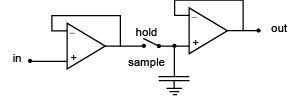Multiplexers

Often several analog signals need to be monitored. If a sufﬁciently fast A/D converter is available, its input can be switched between the various signals using an electronic single­pole multiple­thro w switch.
This device is called a multiplexer. Many ADC chips have built­in multiplexers and S/H circuits.

Differential Ampliﬁers

Often the quantity of interest is the difference between two voltages (e.g. the voltage drop across a resistor) rather than the difference between a signal and ground. The circuit shown below combines an inverting and a non­in verting ampliﬁer into a device called a differential ampliﬁer. This circuit is also useful when an undesired signal (noise) is present on both inputs. Since the differential ampliﬁer only responds to the difference between the two voltages, it will not respond to this common­mode noise.

Low­Pass Filters

Often the signal of interest is changing slowly (e.g. the voltage output of a battery as it discharges) but has an additional higher­frequenc y component superimposed on it (e.g. voltage ﬂuctuations due to a rapidly changing load). A circuit called a low­pass ﬁlter will allow the slowly­changing (low­frequenc y) signal component to pass through to the output while removing or attenuating the fast­changing (highfrequency) component. Filters (low­pass as well as other types) can be built using op­amps, resistors and capacitors.

Offer running on EduRev: Apply code STAYHOME200 to get INR 200 off on our premium plan EduRev Infinity!

159 docs

,

,

,

,

,

,

,

,

,

,

,

,

,

,

,

,

,

,

,

,

,

,

,

,

,

,

,

;